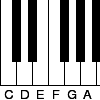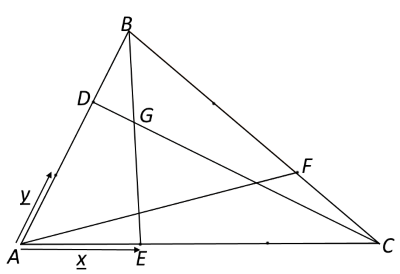#### You may also like### Pent

The diagram shows a regular pentagon with sides of unit length. Find all the angles in the diagram. Prove that the quadrilateral shown in red is a rhombus.### Tuning and Ratio

Why is the modern piano tuned using an equal tempered scale and what has this got to do with logarithms?### Rarity

Show that it is rare for a ratio of ratios to be rational.

# Another Triangle in a Triangle

##### Age 16 to 18 Challenge Level:
An alternative approach is to use vectors.
In the diagram below, vectors $\bf{x}$ and $\bf{y}$ have been defined such that $\overrightarrow{AC}=3\bf{x}$ and $\overrightarrow{AB}=3\bf{y}$.Click below for a series of hints to help you to work out the ratio of the length $DG$ to $DC$:

Can you express $\overrightarrow{DC}$ in terms of $\bf{x}$ and $\bf{y}$?
Can you express $\overrightarrow{BE}$in terms of $\bf{x}$ and $\bf{y}$?

By writing $\overrightarrow{DG}$ as $\lambda \overrightarrow{DC}$ and $\overrightarrow{BG}$ as $\mu \overrightarrow{BE}$, can you find two expressions for $\overrightarrow{AG}$?

By equating coefficients of $\bf{x}$ and $\bf{y}$, can you find $\lambda$?

Now you can use this information together with the first two hints in the problem to find the relationships between the areas.